# Hybrid Orbitals

Last updated: February 20th, 2020 |

Today’s post is all about hybrid orbitals. Here’s a quick summary: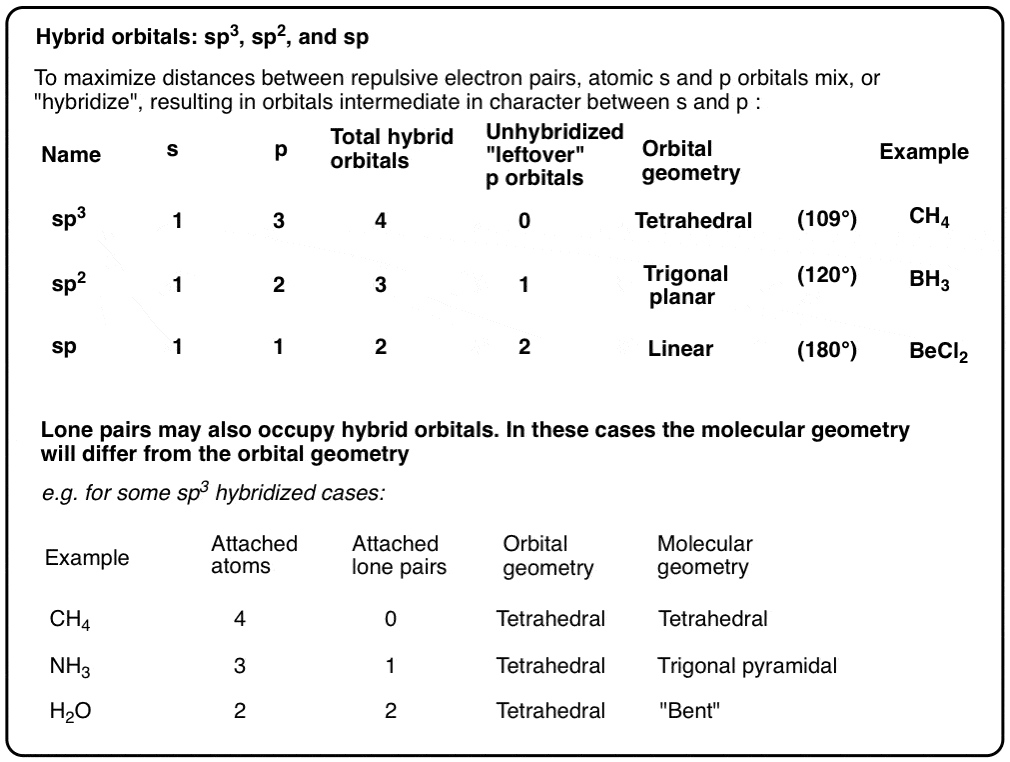[Note: This post was co-authored with Matthew Pierce of Organic Chemistry Solutions.  Ask Matt about scheduling an online tutoring session here. ]

## 1. What Orbitals Are Involved In The Tetrahedral Arrangement Of Methane (CH4)?

In the last post on the structure of methane we asked how we know that methane is tetrahedral.

Based on the orbitals of carbon (2s and 2p) we might have naively expected three of the C-H bonds to line up along the x, y, and z axes, respectively, and have the other one at some arbitrary angle (like 135° or so)

But then, as so often happens in science, our beautiful intuitive hypothesis was destroyed by some annoying experimental facts:

• methane has no measurable dipole moment (our “reasonable” structure, below left, would be expected to have a measurable dipole moment)
• the crystal structure of diamond is tetrahedral, with identical bond lengths and angles between carbons of 109.5 degrees. This isn’t what we’d expect if we were dealing with bonds between “pure” 2s and 2p orbitals!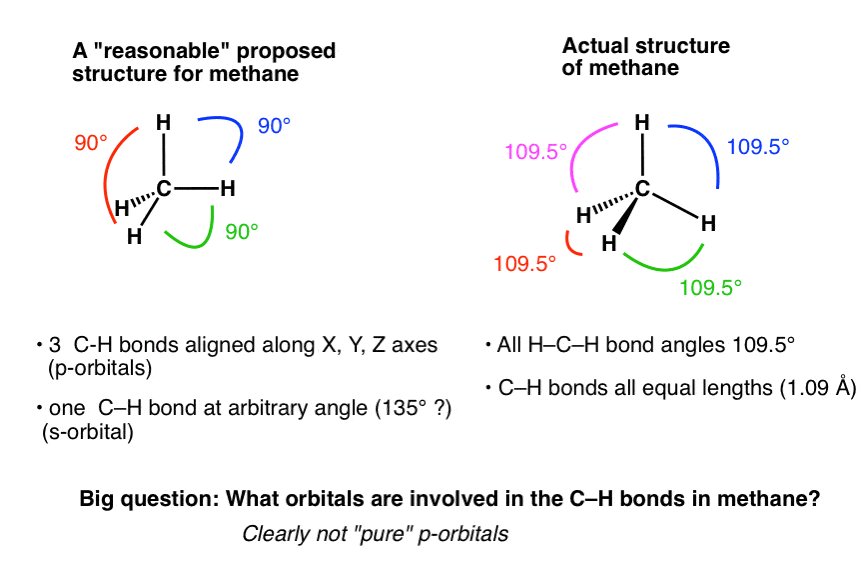In retrospect, the geometry makes sense. It happens that 109.5 degrees is the orientation that maximizes the distance between each of the four bonding pairs, and thus minimizes their repulsive interactions. In other words, the geometry is a direct consequence of “opposite charges attract, like charges repel”.

But how do we describe the orbitals that are used to give that bond angle?

This is a real chin-scratcher. They can’t be pure 2s orbitals (there’s only one 2s orbital, anyway). And they can’t be pure p orbitals, since the p orbitals are aligned at 90° to each other.

So what the heck kind of orbitals are they?

## 2. Hybrid Orbitals: An Explanation For Bonding At Carbon

Linus Pauling asked this same question back in his classic treatise, the Nature of the Chemical Bond (1931) [pdf] which in large measure won him the 1954 Nobel Prize in Chemistry.

Pauling’s solution to this dilemma, which we still apply today, was the following:

• None of the bonding orbitals in methane are 100% s or 100% p. Instead, they are hybrid orbitals that each have partial s character and partial p character.
• The three 2p orbitals and the single 2s orbital hybridize (i.e., mix) to create four hybrid sp3 orbitals, which are arranged tetrahedrally around the central carbon atom.
• Each of the four hybrid orbitals has 25% s-character and 75% p-character.
• In the case of methane, each of these sp3 hybrid orbitals overlaps with a 1s orbital from hydrogen to form the C-H bonds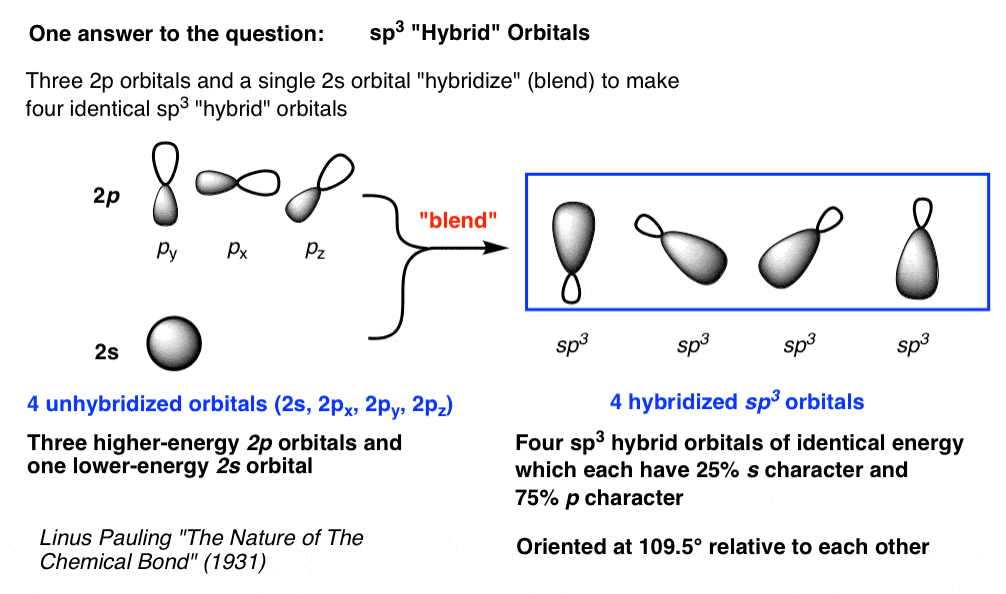I’ll be honest here.  Many students don’t like this explanation.

Certainly, it’s confusing at first.  Why?

I think that when we initially learn about orbitals, we intuitively think of them as containers – sort of like atomic Tupperware for holding electrons that happen to come in a variety of cutesy shapes.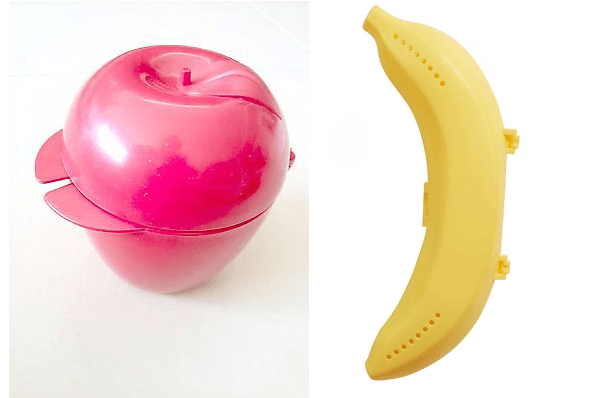Electrons, then,  can be imagined to behave like the “fruits”  that rattle around in these containers (strict limit: 2 per container!). It’s not so surprising to our intuition when we learn that they can move from container to container within an atom or even leave the atom altogether.

What is surprising is when we open up the fridge and find that our cute little sphere- and dumbbell-shaped Tupperware containers  have changed their shape and merged their properties with each other!   This violates our intuition about how containers behave!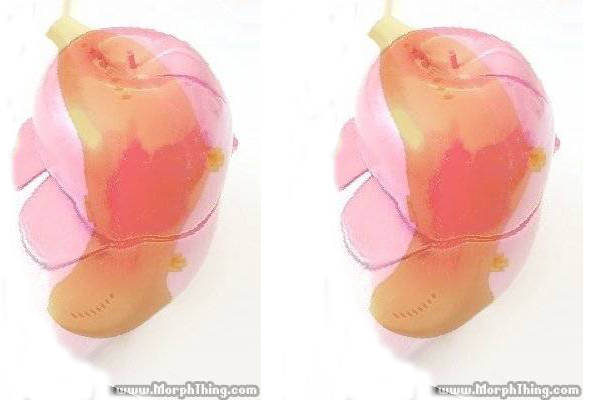This is the quantum world, folks!  If this doesn’t mystify you… it f*&king should!

Going forward, I don’t ask that you understand or even “believe in” hybridization on a deep mathematical or theoretical level. That’s not necessary for our purposes [Pauling was a great teacher. You can always read the original Pauling paper, here, if you choose]

I merely advise that you try to suspend your disbelief going forward, because using this hybridization model will help us rationalize a lot of molecular structure, geometry, and behaviour.

## 3. A Pop Analogy: Hybrid Soda

Before we dive in any further, here’s what I consider to be a helpful little analogy (thx, Steven) that might help to get the point across.

• Imagine you have four bottles of pop: one bottle of Sprite (S)  and three bottles of Pepsi (P).
• Now imagine pouring them out, mixing them all together, and then re-filling each bottle with the mixture.
• The Law of Conservation of Pop says that you still have four bottles worth of liquid. But now the pop is neither pure Sprite or pure Pepsi; it is a hybrid between the two.
• Specifically, each bottle now has 25% Sprite character and 75% Pepsi character.
• We can call this “hybrid” pop, if you like, sp3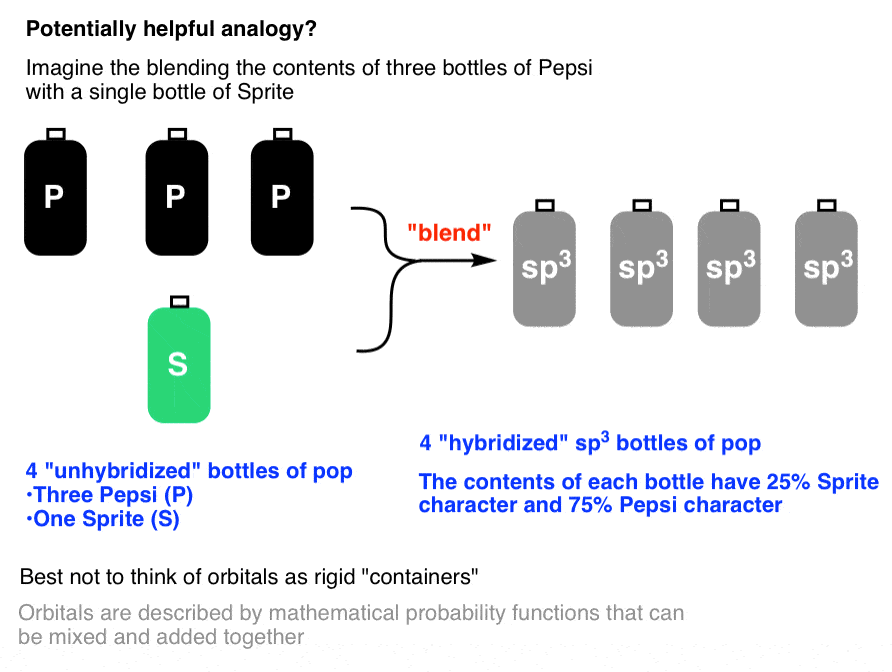That’s a little bit like what has happened to our 2s and 2p orbitals. By mixing the 2s and three 2p orbitals, we now have four orbitals which have 25% s character and 75% p character. (Although, importantly, it’s the shapes of the bottles that are changing, not just the contents).

The final step is to arrange these four orbitals at the corners of a tetrahedron, which allows for the maximum distance between the four electron pairs (like charges repel!)

How does this rationalization help us?

• It explains the tetrahedral molecular geometry of methane, with 4 identical H–C–H bond angles (109.5°)
• It explains the 4 identical C–H bond lengths (and bond strengths) in methane
• It explains the lack of a dipole moment in methane, since the tetrahedral arrangement of the electron pairs allows for all partial charges to cancel (i.e. the vectors sum to zero)
• The model even helps to rationalize how certain reactions occur, which we won’t go into right now  [e.g. backside attack in the SN2 reaction occurs into the empty “antibonding” orbital 180° to the bonding C–H orbital, if you’ve been reading ahead]

## 4. sp3 Hybridization Accurately Describes The Arrangement Of Atoms In (First-Row) Elements Bonded To Four Atoms…

This doesn’t just apply to methane. It applies to any situation where a (first-row) element is bonded to four atoms. Obviously tetrahedral carbon is the most prominent example we will explore, but it also applies to tetrahedral nitrogen [e.g. NH4(+)] and even tetrahedral boron [e.g. BF4(-)].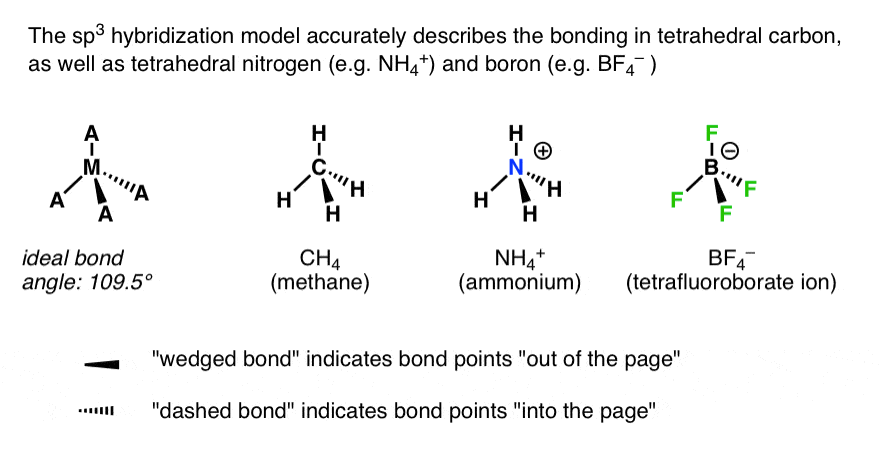## 5….As Well As Situations Where The Number of [Atoms + Lone Pairs] Equals 4

The tetrahedral arrangement of the orbitals holds even when one or more pairs of electrons is a non-bonded lone pair (If you’ve run across VSEPR theory, which most people have by the time that they arrive in organic chem, this shouldn’t come as a shock.)

For instance, ammonia (NH3), the “methyl anion” (CH3 – ) and the hydronium ion (H3O+) all have a central atom with 4 pairs of electrons:  3 pairs of bonding electrons and one pair of non-bonded electrons. As we’ve seen, the ideal geometry for arranging four pairs of electrons  is tetrahedral, which makes the hybridization of the central atom sp3.  This leaves the molecule with a “piano stool” arrangement of atoms about the central atom, which we call “trigonal pyramidal” geometry.

Another way of putting it is that the central atom has tetrahedral orbital geometry (sp3) and trigonal planar molecular geometry.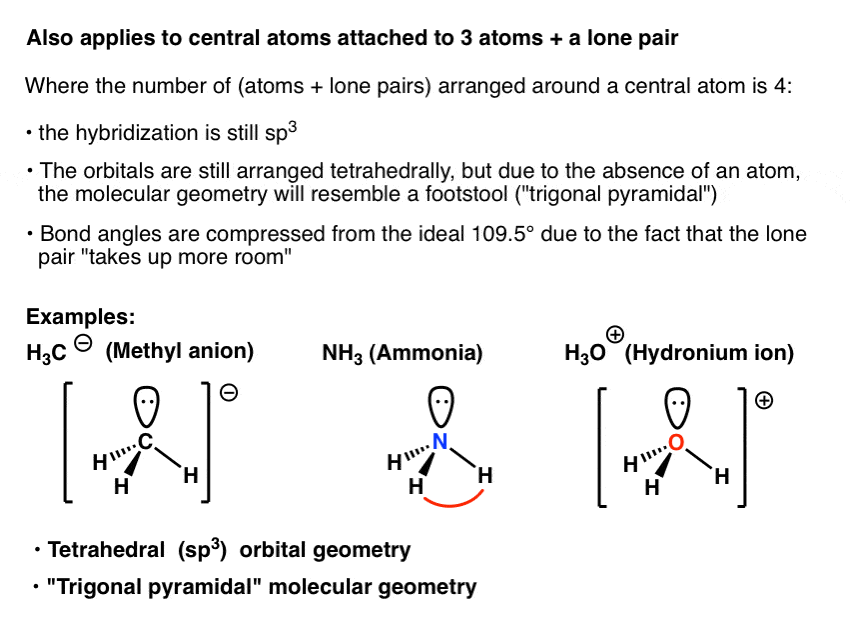An interesting fact is that the bond angles in these are compressed from the ideal angle of 109.5°. The H-N-H bond angles in ammonia, for instance, are 107 degrees. We rationalize this as being due to the fact that a non-bonded lone pair is more repulsive than a “normal” bonding pair – likely because it’s closer to the atom and exerts a stronger influence.

This deviation from “ideal” bond angles is even greater in H2O (water) which has two lone pairs. The hybridization is still sp3, the orbital geometry is still tetrahedral, but the shape (“molecular geometry”) of the resulting molecule is “bent”.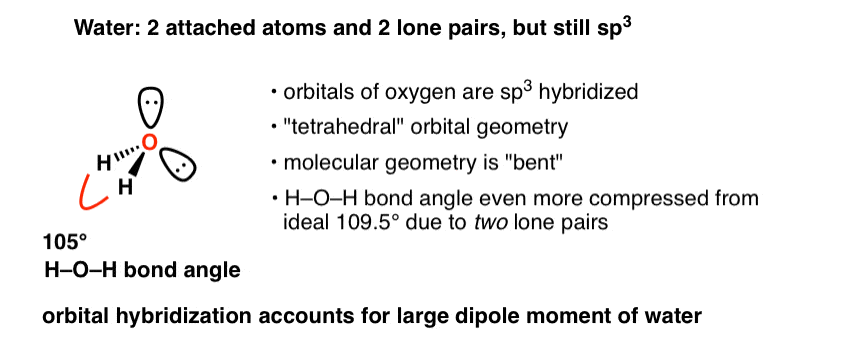Indeed, one of the notable achievements of Pauling’s hybridization model is that it correctly accounts for the dipole moment of water. If water were perfectly “linear”, as many of us might have naively assumed before we learned any chemistry,  the dipoles would cancel each other out.

Another example of “bent” geometry is found in the amide anion NH2 which has two lone pairs on nitrogen.

A quick table might help to summarize everything we’ve established about sp3 hybridization: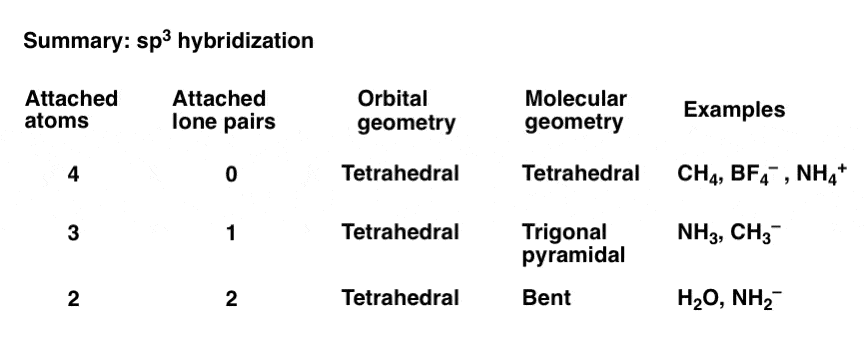## 6. sp2 Hybridization

Let’s go back to our pop-bottle analogy. Say we only mix our Sprite with two bottles of Pepsi, not three.

What does that leave us with?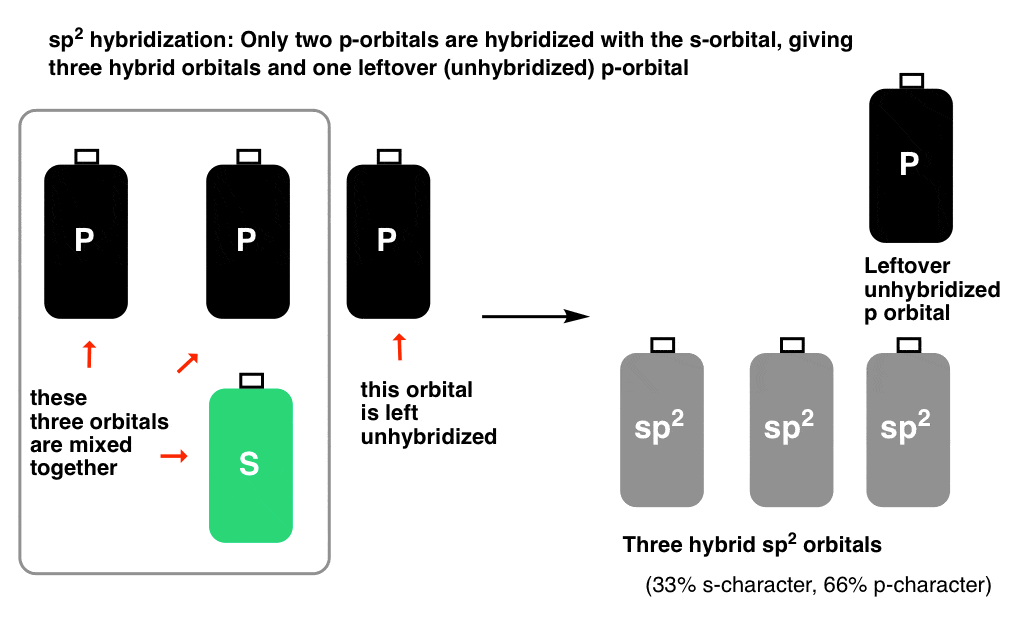This gives us three hybrid bottles of pop, and one leftover unhybridized bottle.

By analogy,  if we mix the 2s orbital with two 2p orbitals, we obtain three sp2 hybrid orbitals, and one left over, unhybridized p orbital.

When these three sp2 orbitals are filled with electron pairs, the bond angle that maximizes their distance apart is 120°.

This gives us a “trigonal planar” arrangement of sp2 orbitals, with the unhybridized p orbital at right angles to the plane. It kind of resembles a Mercedes Benz symbol.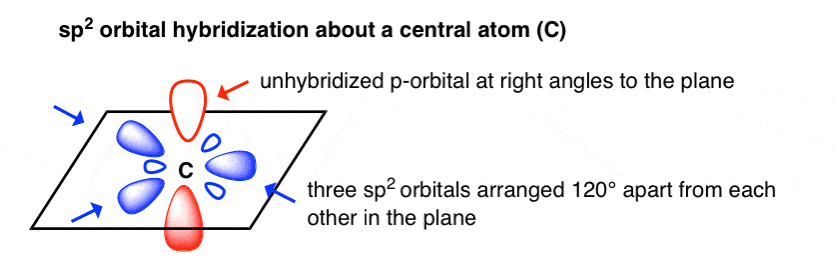A classic example of this trigonal planar geometry is seen in borane, BH, which has three pairs of bonding electrons arranged at 120° to each other.  The trigonal planar geometry is also found in carbocations, such as the methyl cation, CH3.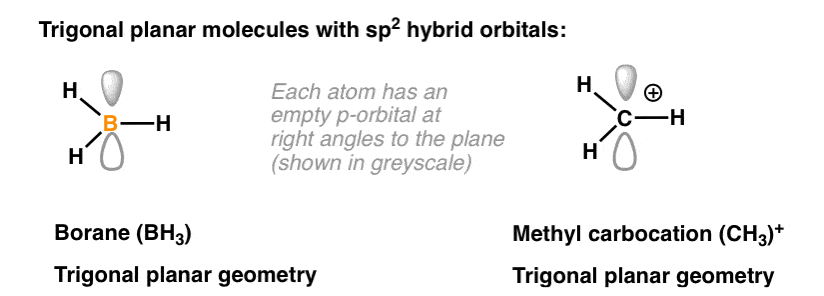You might wonder: where  is the unhybridized p orbital?

At right angles to the plane. Recall that each of the three p orbitals are at right angles to each other. So whichever two p-orbitals hybridize, the third (leftover) p orbital will be at right angles to the plane that they form (just like the z axis is perpendicular to the xy plane)

In the case of BH3 and carbocations, the unhybridized p-orbital is empty.

There is another very common situation where sp2 geometry is observed, however. If adjacent atoms have single electrons in unhybridized p-orbitals, and if those p-orbitals can overlap, a bond can result. This is a phenomenon known as “pi-bonding“.  (We’ll have a lot more to say about it later.)

Pi bonds – which are often just called, “double bonds” – require an unhybridized p orbital in order to form.

The carbon, oxygen, and nitrogen atoms in the examples below, which all have pi bonds (double bonds) are sp2 hybridized. The orbitals are separated by angles of approximately 120°.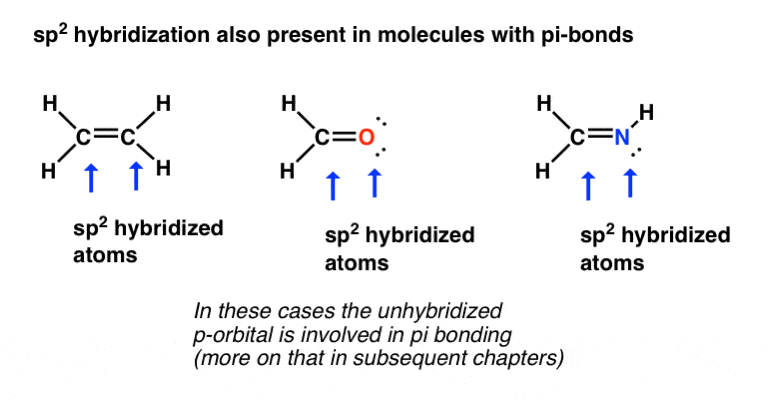Note that lone pairs can be in sp2-hybridized orbitals, just as we saw in NH3 and H2O in the case of sp3 hybridization. Also note that in the midde molecule (formaldehyde), the oxygen has two lone pairs (each in sp2 hybridized orbitals) and in the top right molecule the nitrogen has a single lone pair in a sp2 hybridized orbital.

When a lone pair is present, the bond angles will be slightly less than 120° since the lone pair can be thought of taking up more “room”.

## 7. sp Hybridization

Let’s examine the last possible case. What if only one p orbital hybridizes with the s orbital?

This gives us two hybrid ” sp ” orbitals separated by the maximum angle apart: 180 degrees. We call this arrangement, “linear”. Each hybrid sp orbital has 50% s character and 50% p character.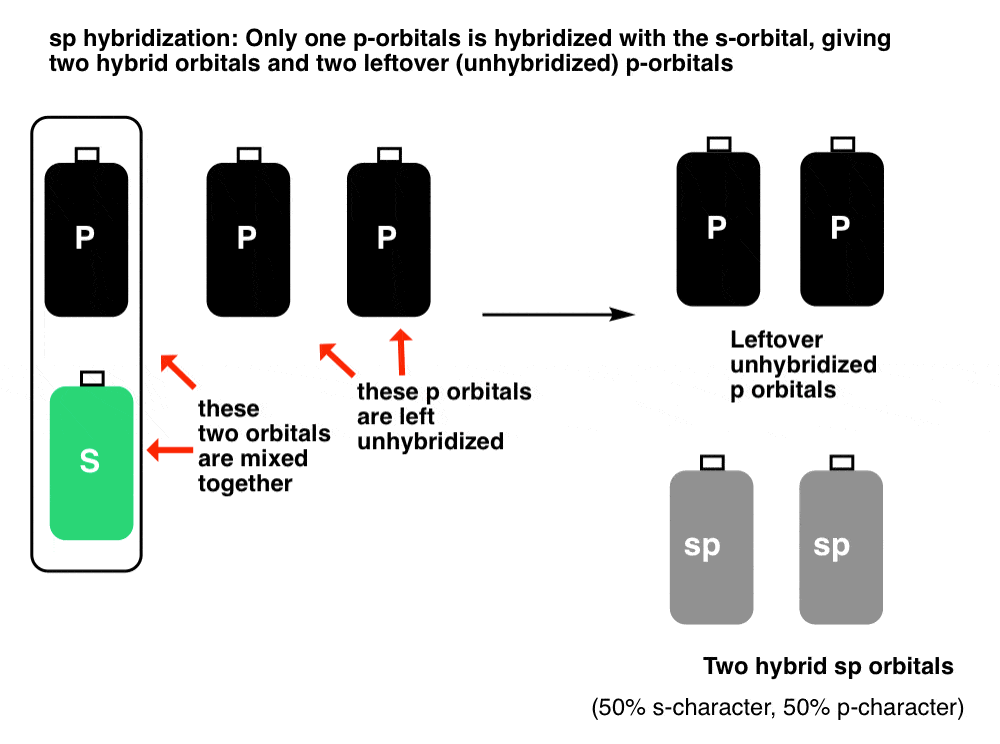The two unhybridized p-orbitals are each at right angles to the sp hybrid orbitals.

For instance, here’s what the orbitals look like in beryllium chloride (BeCl2) where the  Cl-Be-Cl bond angle is 180°. If we consider the Cl-Be-Cl bond to be along the x-axis, the two (unhybridized) p-orbitals will be along the y and z axes, respectively.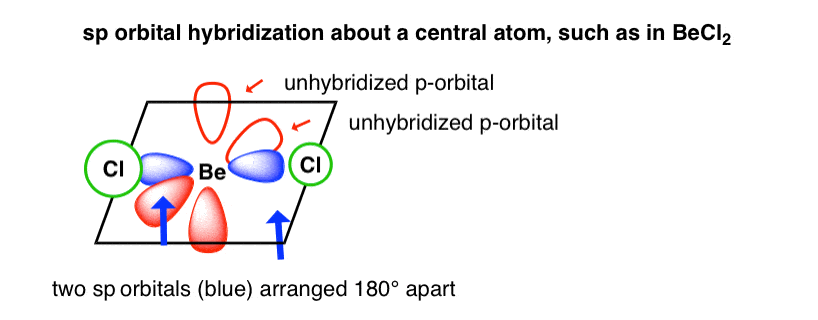sp-hybridization is more commonly observed in situations where there are two pi bonds on a single atom. The most prominent examples are “triple bonds”,  as seen in alkynes, nitriles, and carbon monoxide (CO). In these cases, not only are the carbon atoms sp-hybridized, but so are the nitrogen (in nitriles) and oxygen (in carbon monoxide) atoms.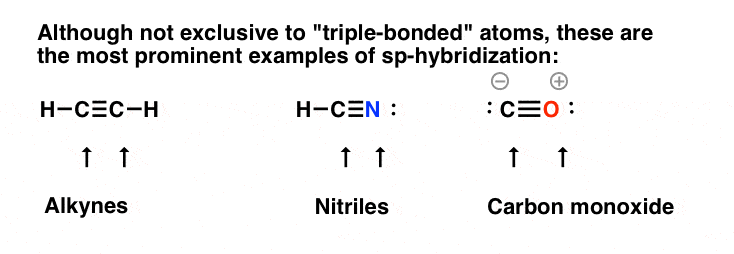Note also that lone pairs can be in sp-hybridized orbitals, as seen in nitriles and carbon monoxide.

sp-hybridization is not exclusive to triple bonded atoms – for example, the central carbons in allene and ketene participate in two pi bonds and are therefore sp-hybridized – but triple bonds are the most immediately recognizable examples.

## 8. Summary – Hybrid Orbitals

OK. Here are the main points of this unintentionally very long post:

• Electron pairs repel. In the absence of orbital hybridization, the bond angles around CH4 would be confined to the geometry of the p-orbitals (90°).  It’s energetically favorable for the s and p orbitals to hybridize to form sp3 orbitals which results in a greater separation of the electron pairs and bond angles of 109° (i.e. at the apices of a tetrahedron).  This also holds for central atoms with non-bonding electron pairs such as NH3 and H2O.
• When only two p orbitals participate in hybridization, three sp hybrid orbitals result that adopt a trigonal planar orbital geometry. The remaining (unhybridized) p orbital, which is at right angles to the trigonal plane,  can either be empty (as in BH3) or singly-occupied (as seen in molecules containing pi bonds).
• When only one p orbital participates in hybridization, the result is two sp hybrid orbitals and a linear orbital geometry. The two remaining p orbitals are available for pi-bonding (as in triply-bonded organic compounds such as alkynes and nitriles) or can be empty (as in BeCl2).
• Note that the number of orbitals around the central atom is always 4. Orbitals are neither created nor destroyed by hybridization; they are merely transformed.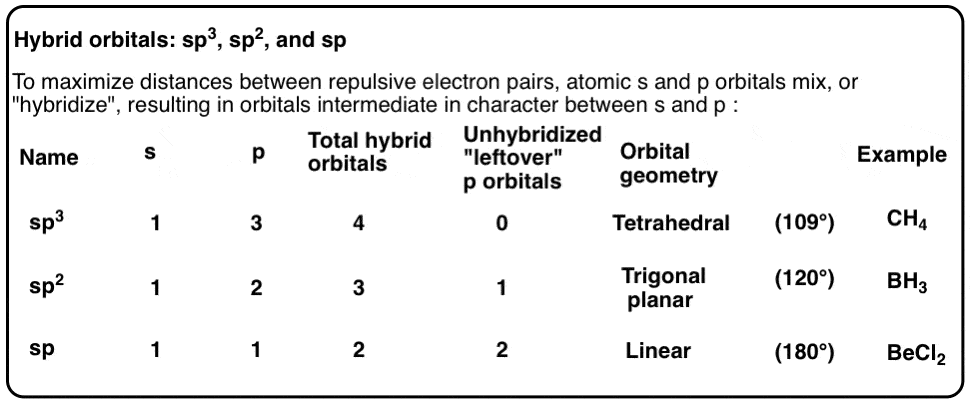In the next post we will just provide a super simple trick for quickly determining the hybridization of a central atom.

Thanks again to Matt for co-authoring. Ask Matt about scheduling an online tutoring session here.

## Comment section

### 2 thoughts on “Hybrid Orbitals”

1.David says:

Thank you James (and also Matt) for another really illustrative post.

Another thing that has always bugged me, which is also related to this topic, is the hybridization of Oxygen (especially explaining the bond angle of 104.5° for water).
I have spoken to a lot of people and professors and it seems that the opinions on this are pretty much split.

On the one hand, there is people saying that Oxygen hybridizes just as Carbon does and that the two lone pairs push the angle from 109.5° to 104.5°.

On the other hand, people claim that the s and p-orbitals of Oxygen are energetically too far apart to hybridize and this model is just an easy but inaccurate explanation.
According to this, the two Hydrogens bond to two p-orbitals at an angle of 90°, but due to repulsion don’t sit exactly on the p-axes but are slightly off (104.5°), resulting in a poorer orbital overlap (unfavorable, but more favorable than hybridization).

A third approach i have heard is bent’s rule (Henry bent), which says that in an sp^n hybrid-orbital, n does not have to be a whole integer. So the orbitals for the lone pairs would have more s-character (because closer to the nucleus) and for the Hydrogens more p-character (because further away from nucleus).

Maybe some day, if you have the time and resources you could discuss this topic.
I would really be interested in your take on this.

Best regards from Austria.

2.Bezverkhniy Volodymyr Dmytrovych says:

The present work shows the inapplicability of the Pauli principle to chemical bond, and a new theoretical model of the chemical bond is proposed based on the Heisenberg uncertainty principle.

See pp. 88 – 104 Review. Benzene on the Basis of the Three-Electron Bond. (The Pauli exclusion principle, Heisenberg’s uncertainty principle and chemical bond). http://vixra.org/pdf/1710.0326v1.pdf

The Pauli exclusion principle and the chemical bond.

The Pauli exclusion principle — this is the fundamental principle of quantum mechanics, which asserts that two or more identical fermions (particles with half-integral spin) can not simultaneously be in the same quantum state.

Wolfgang Pauli, a Swiss theoretical physicist, formulated this principle in 1925 . In chemistry exactly Pauli exclusion principle often considered as a ban on the existence of three-electron bonds with a multiplicity of 1.5, but it can be shown that Pauli exclusion principle does not prohibit the existence of three-electron bonds. To do this, analyze the Pauli exclusion principle in more detail.

According to Pauli exclusion principle in a system consisting of identical fermions, two (or more) particles can not be in the same states . The corresponding formulas of the wave functions and the determinant are given in the reference (this is a standard consideration of the fermion system), but we will concentrate our attention on the derivation: “… Of course, in this formulation, Pauli exclusion principle can only be applied to systems of weakly interacting particles, when one can speak (at least approximately on the states of individual particles) “. That is, Pauli exclusion principle can only be applied to weakly interacting particles, when one can talk about the states of individual particles.

But if we recall that any classical chemical bond is formed between two nuclei (this is a fundamental difference from atomic orbitals), which somehow “pull” the electrons one upon another, it is logical to assume that in the formation of a chemical bond, the electrons can no longer be regarded as weakly interacting particles . This assumption is confirmed by the earlier introduced notion of a chemical bond as a separate semi-virtual particle (natural component of the particle “parts” can not be weakly interacting).

Representations of the chemical bond given in the chapter “The Principle of Heisenberg’s Uncertainty and the Chemical Bond” categorically reject the statements about the chemical bond as a system of weakly interacting electrons. On the contrary, it follows from the above description that in the chemical bond, the electrons “lose” their individuality and “occupy” the entire chemical bond, that is, the electrons in the chemical bond “interact as much as possible”, which directly indicates the inapplicability of the Pauli exclusion principle to the chemical bond. Moreover, the quantum-mechanical uncertainty in momentum and coordinate, in fact, strictly indicates that in the chemical bond, electrons are a system of “maximally” strongly interacting particles, and the whole chemical bond is a separate particle in which there is no place for the notion of an “individual” electron, its velocity, coordinate, energy, etc., description. This is fundamentally not true. The chemical bond is a separate particle, called us “semi-virtual particle”, it is a composite particle that consists of individual electrons (strongly interacting), and spatially located between the nuclei.

Thus, the introduction of a three-electron bond with a multiplicity of 1.5 is justified from the chemical point of view (simply explains the structure of the benzene molecule, aromaticity, the structure of organic and inorganic substances, etc.) is confirmed by the Pauli exclusion principle and the logical assumption of a chemical bond as system of strongly interacting particles (actually a separate semi-virtual particle), and as a consequence the inapplicability of the Pauli exclusion principle to a chemical bond.

1. Pauli W. Uber den Zusammenhang des Abschlusses der Elektronengruppen in Atom mit der Komplexstruktur der Spektren, – Z. Phys., 1925, 31, 765-783.

2. A.S. Davydov. Quantum mechanics. Second edition. Publishing house “Science”. Moscow, 1973, p. 334.

Heisenberg’s uncertainty principle and chemical bond.

For further analysis of chemical bond, let us consider the Compton wavelength of an electron:

λc.е. = h/(me*c)= 2.4263 * 10^(-12) m

The Compton wavelength of an electron is equivalent to the wavelength of a photon whose energy is equal to the rest energy of the electron itself (the standard conclusion is given below):

λ = h/(m*v), E = h*γ, E = me*c^2, c = γ*λ, γ = c/λ

E = h*γ, E = h*(c/λ) = me*c^2, λc.е. = h/(me*c)

where λ is the Louis de Broglie wavelength, me is the mass of the electron, c, γ is the speed and frequency of light, and h is the Planck constant.

It is more interesting to consider what happens to an electron in a region with linear dimensions smaller than the Compton wavelength of an electron. According to Heisenberg uncertainty in this area, we have a quantum mechanical uncertainty in the momentum of at least m*c and a quantum mechanical uncertainty in the energy of at least me*c^2 :

Δp ≥ mе*c and ΔE ≥ me*c^2

which is sufficient for the production of virtual electron-positron pairs. Therefore, in such a region the electron can no longer be regarded as a “point object”, since it (an electron) spends part of its time in the state “electron + pair (positron + electron)”. As a result of the above, an electron at distances smaller than the Compton length is a system with an infinite number of degrees of freedom and its interaction should be described within the framework of quantum field theory. Most importantly, the transition to the intermediate state “electron + pair (positron + electron)” carried per time ~ λc.е./c

Δt = λc.е./c = 2.4263 * 10^(-12)/(3*10^8) = 8.1*10^(-20) s

Now we will try to use all the above-mentioned to describe the chemical bond using Einstein’s theory of relativity and Heisenberg’s uncertainty principle. To do this, let’s make one assumption: suppose that the wavelength of an electron on a Bohr orbit (the hydrogen atom) is the same Compton wavelength of an electron, but in another frame of reference, and as a result there is a 137-times greater Compton wavelength (due to the effects of relativity theory):

λc.е. = h/(me*c) = 2.4263 * 10^(-12) m

λb. = h/(me*v)= 2*π*R = 3.31*10^(-10) m

λb./λc.е.= 137

where R= 0.527 Å, the Bohr radius.

Since the De Broglie wavelength in a hydrogen atom (according to Bohr) is 137 times larger than the Compton wavelength of an electron, it is quite logical to assume that the energy interactions will be 137 times weaker (the longer the photon wavelength, the lower the frequency, and hence the energy ). We note that 1 / 137.036 is a fine structure constant, the fundamental physical constant characterizing the force of electromagnetic interaction was introduced into science in 1916 year by the German physicist Arnold Sommerfeld as a measure of relativistic corrections in describing atomic spectra within the framework of the model of the N. Bohr atom.

To describe the chemical bond, we use the Heisenberg uncertainty principle:

Δx * Δp ≥ ћ / 2

Given the weakening of the energy interaction 137 times, the Heisenberg uncertainty principle can be written in the form:

Δx* Δp ≥ (ћ * 137)/2

According to the last equation, the quantum mechanical uncertainty in the momentum of an electron in a chemical bond must be at least me * c, and the quantum mechanical uncertainty in the energy is not less than me * c ^ 2, which should also be sufficient for the production of virtual electron-positron pairs.

Therefore, in the field of chemical bonding, in this case, an electron can not be regarded as a “point object”, since it (an electron) will spend part of its time in the state “electron + pair (positron + electron)”, and therefore its interaction should be described in the framework of quantum field theory.

This approach makes it possible to explain how, in the case of many-electron chemical bonds (two-electron, three-electron, etc.), repulsion between electrons is overcome: since the chemical bond is actually a “boiling mass” of electrons and positrons, virtual positrons “help” overcome the repulsion between electrons. This approach assumes that the chemical bond is in fact a closed spatial bag (a potential well in the energy sense), in which “boiling” of real electrons and also virtual positrons and electrons occurs, and the “volume” of this potential bag is actually a “volume” of chemical bond and also the spatial measure of the quantum-mechanical uncertainty in the position of the electron.

Strictly speaking, with such a consideration, the electron no longer has a certain energy, momentum, coordinates, and is no longer a “point particle”, but actually takes up the “whole volume” of chemical bonding. It can be argued that in the chemical bond a single electron is depersonalized and loses its individuality, in fact it does not exist, but there is a “boiling mass” of real electrons and virtual positrons and electrons that by fluctuate change each other. That is, the chemical bond is actually a separate particle, as already mentioned, a semi-virtual particle. Moreover, this approach can be extended to the structure of elementary particles such as an electron or a positron: an elementary particle in this consideration is a fluctuating vacuum closed in a certain spatial bag, which is a potential well for these fluctuations.

It is especially worth noting that in this consideration, electrons are strongly interacting particles, and therefore the Pauli principle is not applicable to chemical bond (for more details, see the section “The Pauli Principle and the Chemical Bond”) and does not prohibit the existence of the same three-electron bonds with a multiplicity of 1.5.

The above is easy to demonstrate with the example of a chemical bond of 1 Å length. Then the wavelength of Broglie is written in the form (the length of the chemical bond is L = 2 * Δx):

λ = 2*π*Δx

and the Heisenberg uncertainty ratio takes the form:

Δx * Δp ≥ (ћ * 137 * 2 * π) / 2

from which we get:

L * Δp ≥ ћ * 137 * 2 * π

where L is the length of the chemical bond, and Δp is the quantum mechanical uncertainty of the momentum of each electron in a given chemical bond.
Whence, we obtain a formula for determining the uncertainty of the momentum in a chemical bond:

Δp ≥ (ћ * 137 * 2 * π) / L

Having made the necessary calculations for a length of 1 Å, we obtain:

Δp ≥ (ћ * 137 * 2 * π) / 10 ^ (-10)

Δp ≥ 9.078 * 10 ^ (-22) kg * m / s

That is, the uncertainty in the pulse is greater than me * c

(me * c = 2.73 * 10 ^ (-22) kg * m / s)

(it is clear that the uncertainty of the electron velocity will be greater than the speed of light), which should be based on our assumptions.

See pp. 88 – 104 Review. Benzene on the Basis of the Three-Electron Bond. (The Pauli exclusion principle, Heisenberg’s uncertainty principle and chemical bond). http://vixra.org/pdf/1710.0326v1.pdf

Benzene on the basis of the three-electron bond:

Review. Benzene on the Basis of the Three-Electron Bond. (The Pauli exclusion principle, Heisenberg’s uncertainty principle and chemical bond). http://vixra.org/pdf/1710.0326v1.pdf

1. Structure of the benzene molecule on the basis of the three-electron bond.
http://vixra.org/pdf/1606.0152v1.pdf

2. Experimental confirmation of the existence of the three-electron bond and theoretical basis ot its existence.
http://vixra.org/pdf/1606.0151v2.pdf

3. A short analysis of chemical bonds.
http://vixra.org/pdf/1606.0149v2.pdf

4. Supplement to the theoretical justification of existence of the three-electron bond.
http://vixra.org/pdf/1606.0150v2.pdf

5. Theory of three-electrone bond in the four works with brief comments.
http://vixra.org/pdf/1607.0022v2.pdf

6. REVIEW. Benzene on the basis of the three-electron bond. http://vixra.org/pdf/1612.0018v5.pdf

7. Quantum-mechanical aspects of the L. Pauling’s resonance theory.
http://vixra.org/pdf/1702.0333v2.pdf

8. Quantum-mechanical analysis of the MO method and VB method from the position of PQS.
http://vixra.org/pdf/1704.0068v1.pdf

9. Review. Benzene on the Basis of the Three-Electron Bond. (The Pauli exclusion principle, Heisenberg’s uncertainty principle and chemical bond). http://vixra.org/pdf/1710.0326v1.pdf

Bezverkhniy Volodymyr (viXra):http://vixra.org/author/bezverkhniy_volodymyr_dmytrovych

This site uses Akismet to reduce spam. Learn how your comment data is processed.